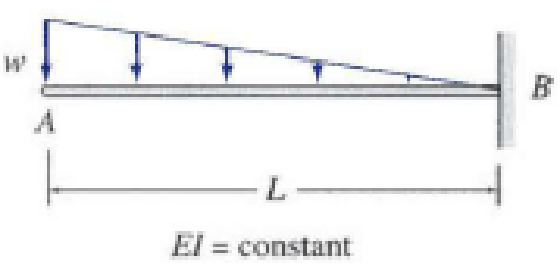# 6.39 Determine the slope and deflection at point A of the beam shown in Figs. P6.13 and P6.14 by the conjugate-beam method. FIG. P6.13, P6.39

#### Solutions

Chapter
Section
Chapter 6, Problem 39P
Textbook Problem
8 views

## 6.39 Determine the slope and deflection at point A of the beam shown in Figs. P6.13 and P6.14 by the conjugate-beam method.FIG. P6.13, P6.39

To determine

Find the slope and deflection of the beam at point A using conjugate-beam method.

### Explanation of Solution

Calculation:

Consider flexural rigidity EI of the beam is constant.

Consider a section at a distance of x from the free end.

Show the section and the free body diagram of the given beam as in Figure (1).

Refer Figure (1),

Determine the intensity of load at x;

wL=wxxwx=wLx

Determine the bending moment at distance x;

Mx=12×wLx×x(L13×x)=12wx2(1x3L)

Since there is no support at point A, there will be no reaction.

Determine the support reaction at B using the relation;

V=0RB12×w×L=0RB=wL2

Consider clockwise moment as negative and counterclockwise moment as positive.

Determine the moment at support B;

+MB=0MB+12×w×L×(23×L)=0MB=wL23

Here, w is the uniformly varying load intensity and L is the distance.

Show the section and M/EI diagram for the given beam as in Figure (2).

Conjugate beam method:

In the real beam A is free end B is a fixed but in conjugate-beam method the end conditions has to be change. Change free end A as fixed end and fixed end B as free.

Show the conjugate beam acted upon by the loading from MEI diagram as in Figure (3).

The slope at A in the real beam is equal to the shear force at A of conjugate beam.

Determine the slope (shear force) at A using the relation;

θA=abwxdx=0Lwx22EI(1x3L)dx

Integrate the equation with respect to x

### Still sussing out bartleby?

Check out a sample textbook solution.

See a sample solution

#### The Solution to Your Study Problems

Bartleby provides explanations to thousands of textbook problems written by our experts, many with advanced degrees!

Get Started

Find more solutions based on key concepts
Present Example 7.1 in Chapter 7 using the format discussed in Section 4.2. Divide the example problem into Giv...

Engineering Fundamentals: An Introduction to Engineering (MindTap Course List)

Design stud anchors for the beam in Problem 9.3-1. Show the results on a sketch similar to Figure 9.11.

Steel Design (Activate Learning with these NEW titles from Engineering!)

What is meant by the term shadow IT?

Principles of Information Systems (MindTap Course List)

What is an entity supertype, and why is it used?

Database Systems: Design, Implementation, & Management

Why would a systems analyst have to act as a translator? What groups might be involved?

Systems Analysis and Design (Shelly Cashman Series) (MindTap Course List)

Describe how desktop monitors, laptop and mobile device screens, smartphones, wearable devices, portable media ...

Enhanced Discovering Computers 2017 (Shelly Cashman Series) (MindTap Course List)

If your motherboard supports ECC DDR3 memory, can you substitute non-ECC DDR3 memory?

A+ Guide to Hardware (Standalone Book) (MindTap Course List)